# Single-event GLM design matrix construction and inference

### Dependencies and setup

This walkthrough uses CANlab object-oriented analysis functions with SPM12 basic functions (e.g., image reading/writing, HRF) in Matlab.
Do you have the relevant tools on your Matlab path?
which create_design_single_event
/Users/torwager/Documents/GitHub/CanlabCore/CanlabCore/Model_building_tools/create_design_single_event.m
If you get an error, you don't! You need the Canlab Core repository. See this page for setup instructions.
You also need the Statistics and Machine Learning Toolbox in Matlab. Try this to check:
if isempty(ver('stats')), warning('You do not have the statistics toolbox on your Matlab path. You may/will get errors'); end

### Stats resources

This lab introduces some of the statistics underlying linear models -- the most common way of analyzing data. Linear models are everywhere in science, business, education, public policy and health, and beyond. The labs assume that you have some basic background knowledge in statistics from an introductory course, and dive into some important aspects of creating and understanding fMRI models. However, learning statistics is an iterative process! There is so much to understand in statistics, and people can often feel lost because many of concepts are not intuitive at first, and require new ways of thinking. My professors told me "you have to learn it three times before it sinks in". This is true, but even beyond that, you can always go back and deepen your understanding or make new connections.
This section has some other statistics resources that might help you to go back and refresh or fill in holes so that you can understand this lab sequence better, or deepen your knowledge for the future. One great resource is Jeannette Mumford's crash course in statistics. She is a wonderful teacher and watching her videos will provide an additional explanation of the core concepts needed to understand the GLM. Like statistics in general, learning neuroimaging is an iterative process. In the beginning, it is important to get a broad understanding of the key steps and how neuroimaging can be used to make inferences, but as you progress in your training you will have plenty of opportunities to zoom into specific steps to learn more about particular details and nuances that you may not have fully appreciated the first time around.

### Conceptual foundation: A simple experimental design

Let's say that we're examining brain responses to famous faces. We'll construct one of the simplest possible designs, by presenting a face for 3 seconds every 18 sec during fMRI scanning.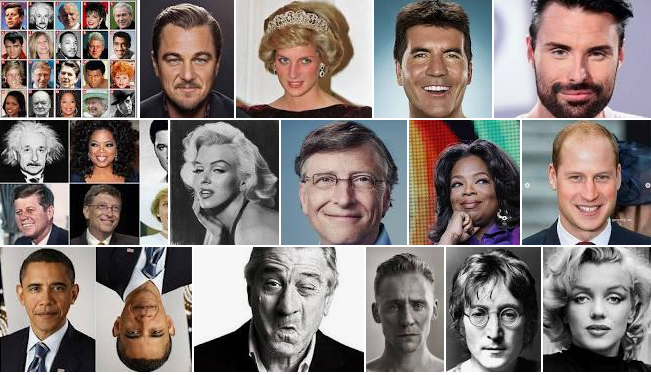We're going to construct a model (X), which is the predicted fMRI signal in a brain area that responds to faces, and fit it to the data. We can use the same model to estimate the amplitude of the response to faces (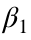) in any voxel or region of a single person's brain (y). Fitting the model involves solving the equation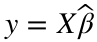, where y is a brain time series (a vector), Xis the model or "design matrix", and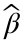is the unknown vector of regression slopes or "parameters".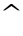or "hat" means something that is estimated from the data during model fitting.
In our examples here, the regression slopes are equivalent to amplitudes for different psychological conditions. In this first, simplest example, we only have one regressor, corresponding to the "face" condition. There are only two model parameters: one for the "face" regressor of interest () and an intercept (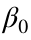). Xis a [T x 2] matrix, where T is the number of time points (fMRI images) collected. It has two columns, an intercept (with constant values for every time point) and a "face" regressor whose values are the predicted rise and fall of a hypothetical "face processing" region across time.is a [2 x 1] vector consisting of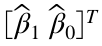.
We can also estimate the amplitude for every voxel in the brain and make a brain map (or β map, beta map) out of them. For now, let's consider just a single brain response variable for a single hypothetical brain time series - for example, the average signal in a person's pre-identified "fusiform face area":